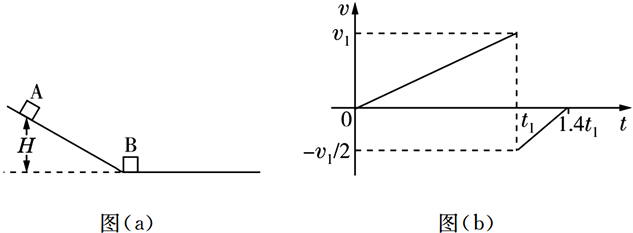# 基于SOLO分类法的试题评价分析——以一道高考物理题为例Analysis on the Evaluation of Test Questions Based on SOLO Taxonomy—Take a Physics Question of College Entrance Examination as an Example

DOI: 10.12677/ASS.2020.97133, PDF, HTML, XML, 下载: 33  浏览: 85

Abstract: Scientific and reasonable evaluation of the physics questions in the college entrance examination is helpful to improve the teaching practice of physics in senior high school. SOLO classification evaluation method is a qualitative evaluation method based on grade description. In this paper, SOLO classification evaluation method is used to evaluate a physics test in college entrance ex-amination, so as to help improve students’ problem-solving thinking ability.

1. 引言

2. SOLO分类理论

2.1. SOLO分类理论基础

SOLO分类(SOLO taxonomy)，其中SOLO (structure of the observed learning outcome)是英文首字母缩写，原意是“观察到的学生学习结果的结构”。是由Biggs和Collis在1982年提出的一种以等级描述为基本特征的质性评价方法 。

Biggs和Collis发现，人的认知水平在总体上和具体事件的执行过程中都具有阶段性，而且这些特征可以通过比较、测试等检测手段观察到，并将学生的学习结果按照思维从低到高分为五个不同水平，分别为前结构水平、单一结构水平、多元结构水平、关联结构水平以及扩展抽象结构水平 。本文参考本山丽娟等人的划分方法，得到非实验题的SOLO层次划分标准 ，如表1所示。Table 1. SOLO Level division criteria for non-experimental questions

2.2. SOLO理论应用于试题评价研究中的优势

3. 高考试题范例分析

1) 求物块B的质量；

2) 在图所描述的整个运动过程中，求物块A克服摩擦力所做的功；

3) 已知两物块与轨道间的动摩擦因数均相等，在物块B停止运动后，改变物块与轨道间的动摩擦因数，然后将A从P点释放，一段时间后A刚好能与B再次碰上。求改变前面动摩擦因数的比值。Figure 1. Title example

3.1. 选题分析

3.2. 评价分析

3.2.1. 前结构水平

3.2.2. 单一结构水平

3.2.3. 多元结构水平

3.2.4. 关联结构水平

3.2.5. 抽象拓展水平

3.3. 最高水平解题赏析

1) 设物块B的质量为 ${m}_{\text{B}}$，碰撞后物块A的速度为 ${v}_{\text{A}}$ ；物块B速度为 ${v}_{\text{B}}$

$m{v}_{1}=m{v}_{\text{A}}+{m}_{B}{v}_{\text{B}}$ (1)

$\frac{1}{2}m{v}_{1}^{2}=\frac{1}{2}m{v}_{\text{A}}^{2}+\frac{1}{2}{m}_{\text{B}}{v}_{\text{B}}^{2}$ (2)

${v}_{A}=-\frac{{v}_{1}}{2}$ (3)

${m}_{\text{B}}=3m$ (4)

$0~{t}_{1}$ 时，物块A由静止运动到斜面底端，此过程重力势能转化为物块A的动能以及物块克服摩擦力所做的功。

${t}_{1}~1.4{t}_{1}$ 时，物块A沿着轨道上滑：此时物块A的动能转化为物块A的重力势能以及克服摩擦力所做的功。

$mgH-f{s}_{1}=\frac{1}{2}m{v}_{1}^{2}-0$ (5)

$-f{s}_{2}-mgh=0-\frac{1}{2}m{\left(-\frac{{v}_{1}}{2}\right)}^{2}$ (6)

${s}_{1}=\frac{1}{2}{v}_{1}{t}_{1}$ (7)

${s}_{2}=\frac{1}{2}×\frac{{v}_{1}}{2}×\left(1.4{t}_{1}-{t}_{1}\right)$ (8)

$\frac{{s}_{2}}{{s}_{1}}=\frac{h}{H}=5$ (9)

$W=f{s}_{1}+f{s}_{2}$ (10)

$W=\frac{2}{15}mgH$ (11)

(3)设轨道倾角为 $\theta$，改变前物块与轨道的动摩擦因素为 $\mu$，改变后物块与轨道的动摩擦因素为 ${\mu }^{\prime }$，p点的高度为h，物体B在水平轨道上滑行距离为 ${s}^{\prime }$

$f=\mu mg\mathrm{cos}\theta$ (12)

$W=\mu mg\mathrm{cos}\theta \cdot \frac{H+h}{\mathrm{sin}\theta }=\frac{2}{15}mgH$ (13)

$-\mu {m}_{\text{B}}g{s}^{\prime }=0-\frac{1}{2}{m}_{\text{B}}{v}_{\text{B}}^{2}$ (14)

$mgh-{\mu }^{\prime }mg\mathrm{cos}\theta \cdot \frac{h}{\mathrm{sin}\theta }-{\mu }^{\prime }mg{s}^{\prime }=0$ (15)

$\frac{\mu }{{\mu }^{\prime }}=\frac{\text{11}}{\text{9}}$ (16)

4. 启示与思考

4.1. 依照思维水平，确定教学任务

1) 首先教师要引导学生迅速获取有用的题干信息，从单点到多点对题目中所需要用到物理物理定律进行简要的分析，从而达到SOLO结构层次的多点水平。

2) 将题干所呈现的图表信息、物体受力方式、所遵循的物理规律与学生已有的知识结构联系起来，找到各个变量、图表之间的关系，从而达到SOLO结构层次的关联水平。

3) 教师在原有解答的基础上要进行适当地拓展，培养学生灵活应对物理试题的能力，达到SOLO结构的抽象拓展水平。比如经典力学中的很多问题可以用牛顿运动定律和能量守恒观念来解决，而运用能量守恒观念解题要比运用牛顿定律更加简便。因此教师在教学过程中要积极培养学生运用能量守恒观念来解决物理问题的意识。同时教师要引导学生多角度分析并解决物理问题，学生也能在使用多种解法求解的过程中总结出适合自己的解题思路。

4.2. 遵循认知规律，分层设置考题

4.3. 分析个性特征，准确因材施教

1) 对于处于前结构水平的学生，他们对于物理试题处于茫然且无从下手的状态，这时教师首先应该树立学生学习物理的兴趣与信心。从基础概念开始有耐心地帮助学生们重构物理知识体系，找回学习物理知识的动力与决心。

2) 对于处于单点结构与多点结构水平的学生，他们在对于物理概念的理解仍然处于量的阶段，不懂得将概念与题干信息以及图表信息进行整体的分析。在彼格斯教授所做的研究表明，那些喜欢熟记事实细节并使用机械学习策略的学生在传统的测试中获得了高分，但他们却同时获得了较低的SOLO等级 。可见靠盲目死记硬背物理公式的学习方式不仅不能效提高学生的思维水平，而且容易导致学生物理知识点的混淆。这就要求教师在深度理解教学内容的基础上，优化教学梯度设计，引导学生从低阶走向高阶的思维水平，实现质的跃迁。

3) 对于处于关联结构水平的学生，他们缺乏的是多角度分析思考问题的能力，这时教师应该鼓励学生鼓励学生积极进行发散性思考，最大限度地发挥其创造性以及创新性，从而引导学生从关联结构水平提升至扩展抽象结构水平，实现质的飞跃。

4.4. 根据层次标准，分层评价作业

SOLO分类评价理论作为一种新型的质性评价方式，将其应用于课后作业批改，可以让教师更加清楚地了解班级学生所处的能力结构，明确评讲作业时的重点以及难点，以此来提高课堂效率。同时学生根据教师评价更能明确自身能力结构水平，激励自己提高到更高层次能力水平。所以利用SOLO分类评价理论对学生的作业进行分析评定，诊断学生的学习情况以及所处的思维结构水平，搭建学生与教师之间反馈的桥梁，能够有效提高作业的针对性、有效性、合理性。

  赵星宇. 基于SOLO分类理论对高中物理作业分析——以牛顿运动定律部分试题为例[J]. 物理通报, 2020(S1): 121-123.  王振超, 李建彬, 胡象岭. 基于SOLO分类理论的高考物理试题能力层次分析——以2016年全国卷Ⅰ为例[J].物理教师, 2018, 39(2): 86-91.  吴有昌, 高凌飚. SOLO分类法在教学评价中的应用[J]. 华南师范大学学报(社会科学版), 2008(3): 96-97.  山丽娟. 高考物理试卷能力结构研究——将SOLO理论运用于试题内容分析初探[D]: [硕士学位论文]. 上海: 华东师范大学, 2011: 9-10.  周甜, 陈建华. 基于SOLO分类法的试题评价研究——以一道高考数学题为例[J]. 高中数学教与学, 2019(9): 4-6.  彼格斯, 科利斯. 学习质量的评价:SOLO分类理论[M]. 高凌飚, 张洪岩, 主译. 北京: 人民教育出版社, 2010: 18-35.